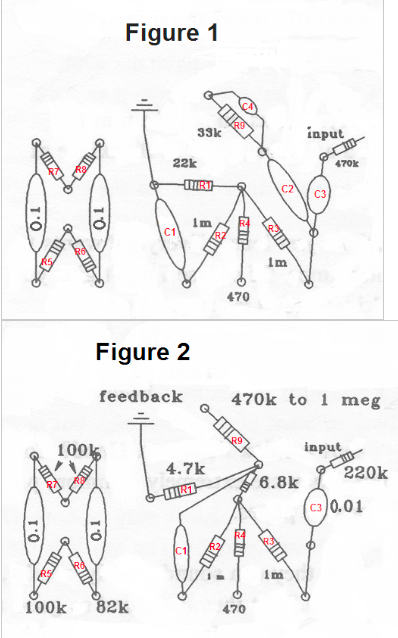## For this project I was given a set of technical instructions that were unclear and were missing information. It was my assignment to research the subject material and find sources that could inform me about how to perform the technical task. Then I used that information to create a new set of clearer and more accurate instructions.

Instructions for Converting to Guitar Amp

Instructions for converting a Tolex Bassman head so that it will put the feedback into the driver tube’s cathode circuit and for rebuilding the cathode circuit to a stronger, more musically sensitive circuit.

Before Starting:

• Make sure that your amp’s circuits look like the drawing in Figure 1
• Check that the amp is working
• Make a list of all of the parts you will need
• 1 meg Resistor (2)
• 6.8k Resistor (1)
• 4.7k Resistor (1)
• 100k Resistor(1)
• 82k Resistor (1)
• 0.1 Capacitor (1)
• 0.01 Capacitor (1)
• Soldering Equipment

IN ORDER TO AVOID ELECTRIC SHOCK
• Unplug amp before starting work
• Discharge filter capacitors before starting work
• Do not work on amp unless you know what you are doing

Instructions

(Figure 1 on the following page demonstrates how the amp looks before the modifications and Figure 2 shows how the amp will look after.)

1. Begin by removing the three 0.1 mfd caps (labeled as C1, C2, and C3 on Figure 1).
2. Remove the 330k resistors and replace both with 1 meg resistors (remove R2 and R3 – Figure 1).
3. Leave the center resistor as is (R4 – Figures 1 and 2).
4. Connect a 6.8k resistor to both new resistors and the old resistor (will look like R2 and R3 – Figure 2).
5. Replace the 22k resistor (R1 – Figure 1) with a 4.7k resistor (R1 – Figure 2).
6. Replace the 0.1 mfd capacitor with a 0.01 capacitor (C3 – Figures 1 and 2).
7. Begin working on the left side of the circuit.
8. Remove the 47k resistors (R5 and R6 – Figure 1)
9. Replace R5 (Figure 1) with a 100k resistor (R5 – Figure 2).
10. Replace R6 (Figure 1) with an 82k resistor (R6 – Figure 2).
11. Make sure that the R7 and R8 resistors (Figures 1 and 2) are 100k resistors.